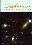© CAMBRIDGE UNIVERSITY PRESS 1997

1.3 Properties of Quasars

By late 1964, Schmidt had studied a sufficient number of quasars to define their properties (Schmidt 1969):

• Star-like objects identified with radio sources.
• Time-variable continuum flux.
• Large UV flux.
• Large redshifts.

Not all objects that we now call AGNs share every one of these properties. In fact, the single most common characteristic of AGNs is probably that they are all luminous X-ray sources (Elvis et al. 1978). Nevertheless, the characteristics identified by Schmidt are important for understanding the physics of AGNs and as well as understanding the techniques by which AGNs are found. We will therefore discuss these various properties individually below.

In more modern terms, one of the defining characteristics of quasars is their very broad spectral energy distribution, or SED (Fig. 1.3). Quasars are among the most luminous objects in the sky at every wavelength at which they have been observed. Unlike spectra of stars or galaxies, AGN spectra cannot be described in terms of blackbody emission at a single temperature, or as a composite over a small range in temperature. Non-thermal processes, primarily incoherent synchrotron radiation, were thus invoked early on to explain quasar spectra.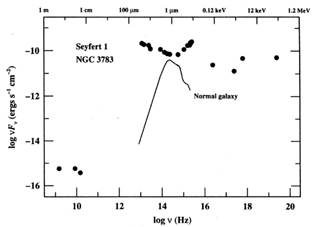Figure 1.3. The spectral energy distribution (SED) of the Seyfert 1 galaxy NGC 3783 (Alloin et al. 1995) from radio to-ray energies. Shown for comparison is SED for a normal (type Sbc) galaxy, from a template spectrum compiled by Elvis et al. (1994). The flux scale of the normal galaxy spectrum has been adjusted to give the correct relative contribution of AGN component and starlight for NGC 3783 (in mid-1992) at 5125 Å through a 5" x 10" spectrograph aperture.

In general, the broad-band SED of a quasar continuum can be characterized crudely as a power law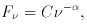(1.5)

whereis the power-law index, C is a constant, and F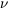is the specific flux (i.e., per unit frequency interval), usually measured in units of ergs s-1 cm-2 Hz-1. The convention that we adopt throughout this book is that a positive spectral index characterizes a source whose flux density decreases with increasing frequency. The reader is cautioned that some authors absorb the minus sign into the definition of the spectral index (e.g., F= C). Specific fluxes, particularly in the ultraviolet and optical parts of the spectrum, are often measured per unit wavelength interval (i.e., in units of ergs s-1 cm-2 Å-1) rather than per unit frequency interval. The total flux measured in any bandpass is the same, of course, whether the bandwidth is measured in frequency or in wavelength, so the relationship Fd= Fdalways holds. The transformation between the two systems is thus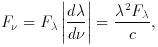(1.6)

so an equivalent form to eq. (1.5) is F= C'-2.

Fits to quasar spectra over large frequency ranges yield spectral indices that are typically in the range 0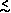1, but different values ofare found for different spectral ranges. Indeed, it is obvious that a single power law cannot hold over all frequencies as the integrated power would diverge either at high frequencies (for1) or at low frequencies (for1). Over a frequency range1 -2, the total power received is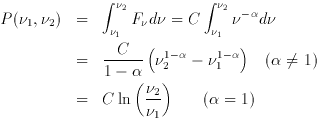(1.7)

The case= 0, a ``flat spectrum'' on a conventional plot of specific flux versus frequency, has equal energy per unit frequency interval and the case= 1 has equal energy per unit logarithmic frequency interval 1. A useful way to plot the broad-band SED in quasars is on a logFversus logdiagram, as in Fig. 1.3. In this case, the power-law distribution becomesF1-, so the case= 1 is a horizontal line in such a diagram, and the= 0 spectrum rises with frequency. This is the preferred format for examining where the quasar energy is actually emitted as it reflects the amount of energy emitted in each equally spaced interval on the logarithmic frequency axis.

1 The case= 1 is sometimes described as having equal energy per decade (or octave), i.e., over any factor of 10 (or two) in frequency. Back.